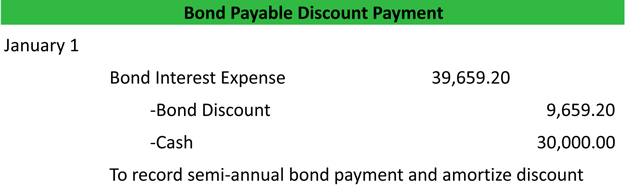# What is the Effective Interest Method?

Definition: The effective interest method is a way of allocating interest expense from a bond evenly and consistently over the life of the bond. Remember when dealing with bonds, there are two different interest rates to deal with: the stated rate that appears on the bond and the market rate. The market rate is the rate of interest that the market is willing to pay for the bond at that time. Since bonds can take a few months to actually get issued to the investors after they are written, the stated rate and the market rate are rarely the same. This is what causes problems.

## What Does the Effective Interest Method Mean?

If a straight line method were used to allocate interest expense, the bond interest expense would be equal for every single payment. You might ask what is wrong with that? Well, the semi-annual interest expense dollar amount will remain equal, but the percentage in relation to the bond will change. For instance, as the bond payments are made, interest is being expensed and the bond premium or discount is reducing or increasing the loan balance. If the interest expense dollar amount stayed the same each payment, the percentage of interest would change.

## Example

Let’s look at an example. A \$100,000 bond is being paid off in \$5,000 semi-annual payments. The bond is being discounted \$500 a payment. On the straight line method, \$4,500 would go to interest each payment like this. As you can see, each payment reduces the bond payable account, but the interest expense stays the same. Thus, the interest expense is becoming a greater portion of bond payable account.

The Effective Interest Method corrects this problem by allocating interest expense to the bond payable each payment. That way the bond interest expense is always equal to the market interest rate of return. Let’s look at an example.

## Bond Discount Amortization Schedule Example

Fretless Guitar, Inc. issues a \$500,000 bond that is to be paid in semi-annual payments over the course of 10 years. The stated rate on the bond is 6% and the market rate is 12%. Both of these interest rates are shown in semi-annual terms. The payment amount will be \$30,000 = (500,000 x .06). Here is an amortization schedule to help calculate the effective interest method of for allocating the bond interest expense for each payment.Download this accounting example in excel to help calculate your own Bond Discount problems.

As you can see, the bond interest expense is always 12% of the carrying value of the bond and the actual cash paid each payment is always equal to the stated rate on the bond or 6%. Here is an example of the first journal entry to record the bond discount and interest expense on the effective interest method.

## Bond Discount Payment Journal Entry Example